# Concise Selina Solutions for Class 9 Maths Chapter 25 - Complementary Angles

Selina Solutions are useful for students as it helps them in scoring high marks in the examination. The Selina Solutions given in this page contains detailed step-by-step explanations to all the problems that come under Chapter 25, Complementary Angles, of the Class 9 Selina Textbook. This chapter is a continuation of Chapter 23 and 24 of class 9 Selina book. This chapter deals with the concept of Complementary Angles.

These solutions are prepared by subject matter experts at BYJU’S, describing the complete method of solving problems. By understanding the concepts used in Selina Solutions for Class 9 Maths, students will be able to clear all their doubts related to “Complementary Angles” of triangles.

### Download PDF of Selina Solutions for Class 9 Maths Chapter 25:-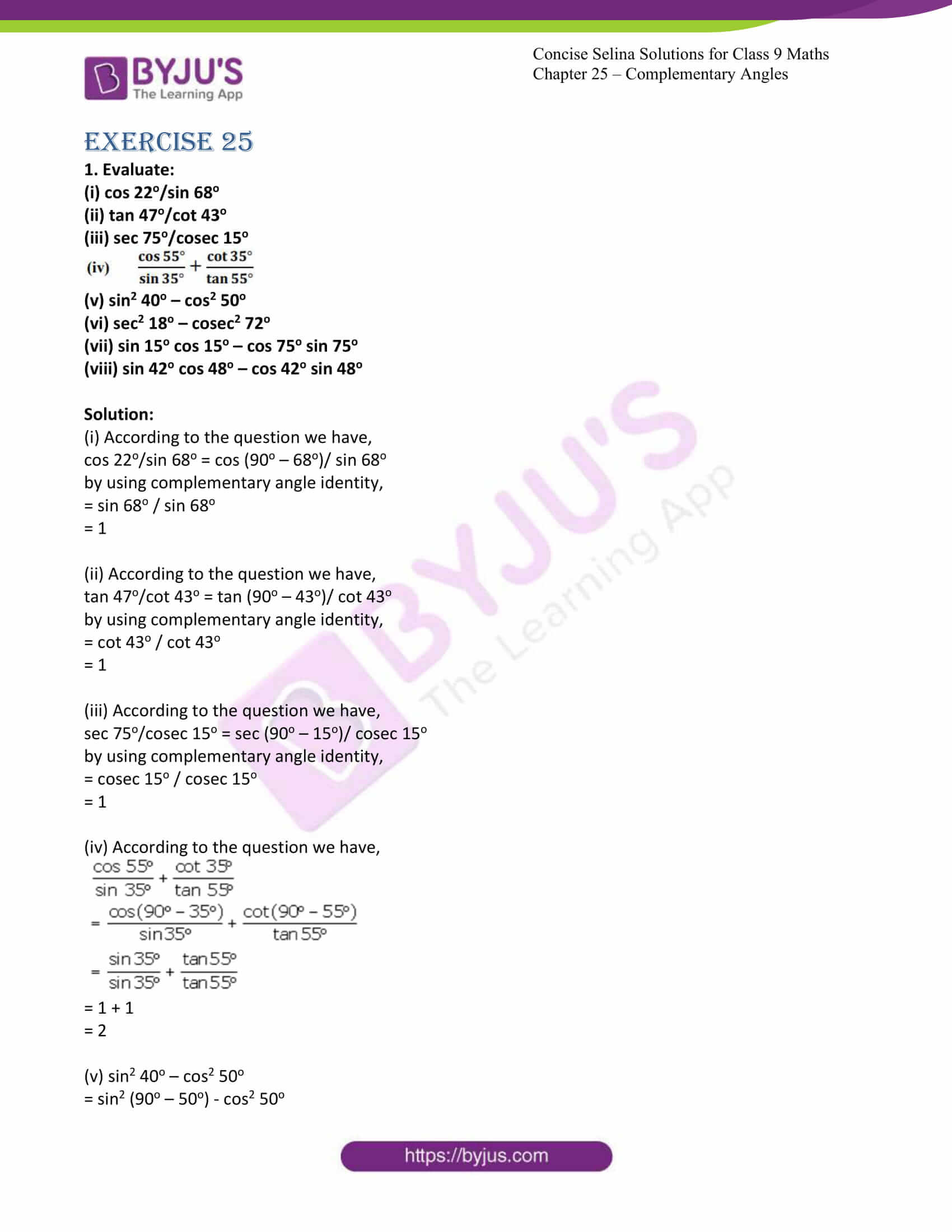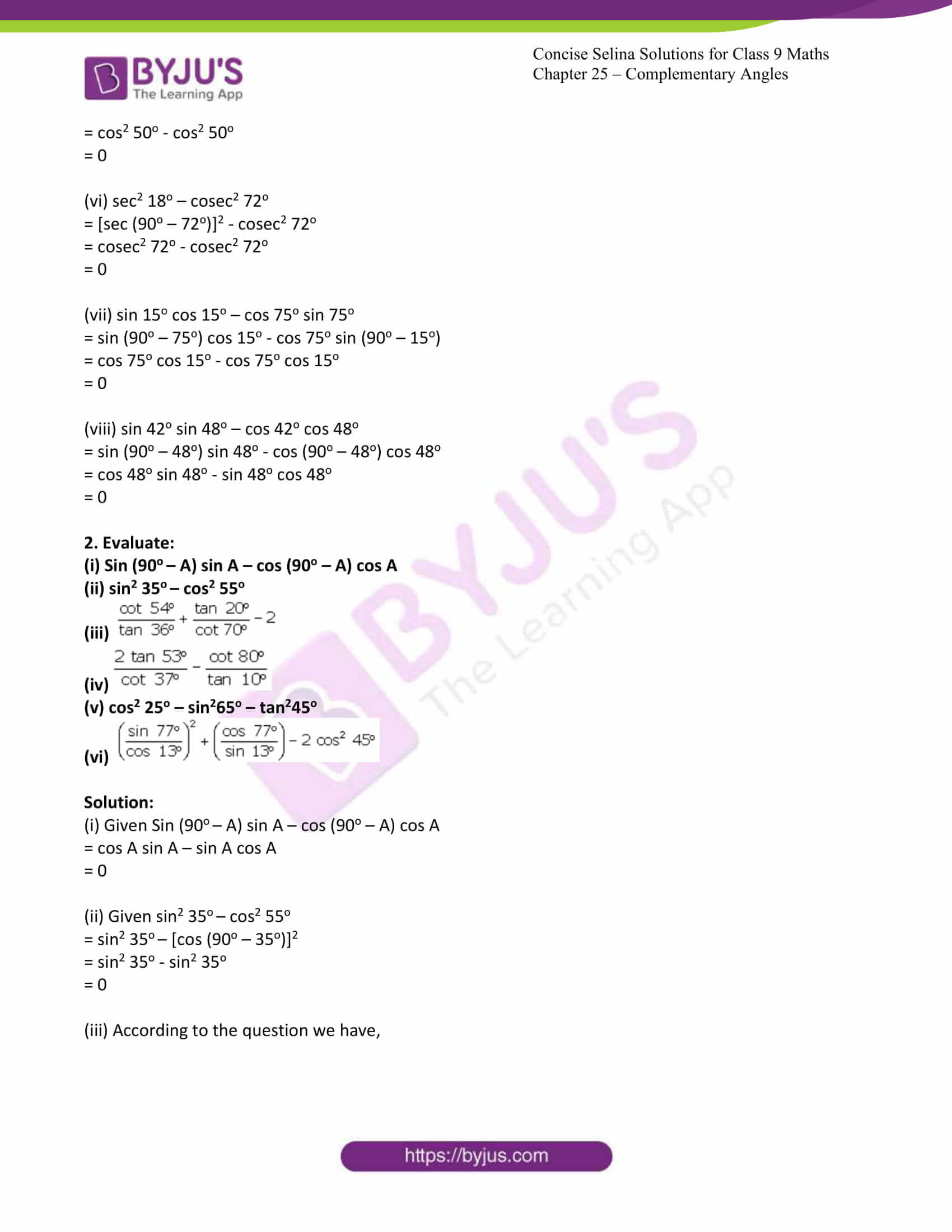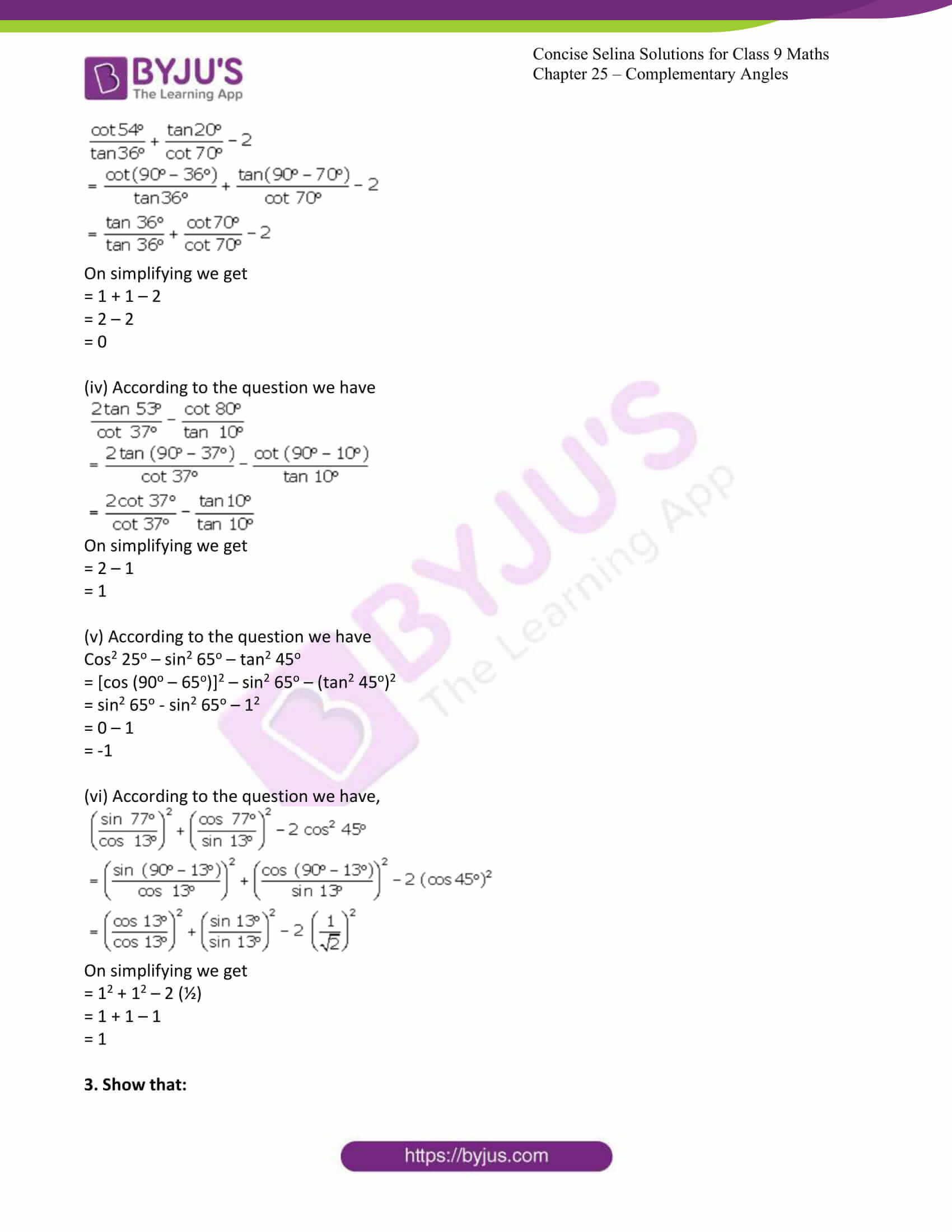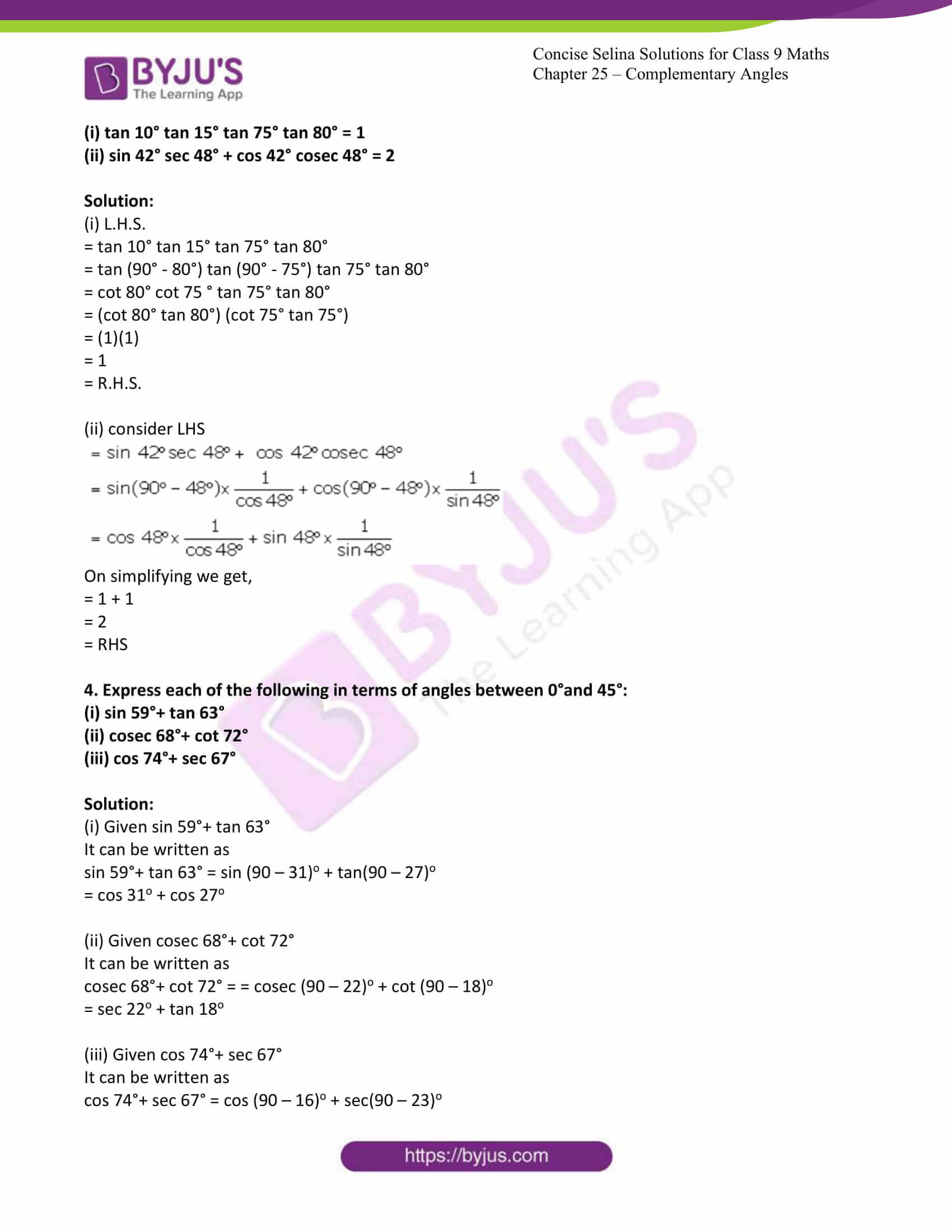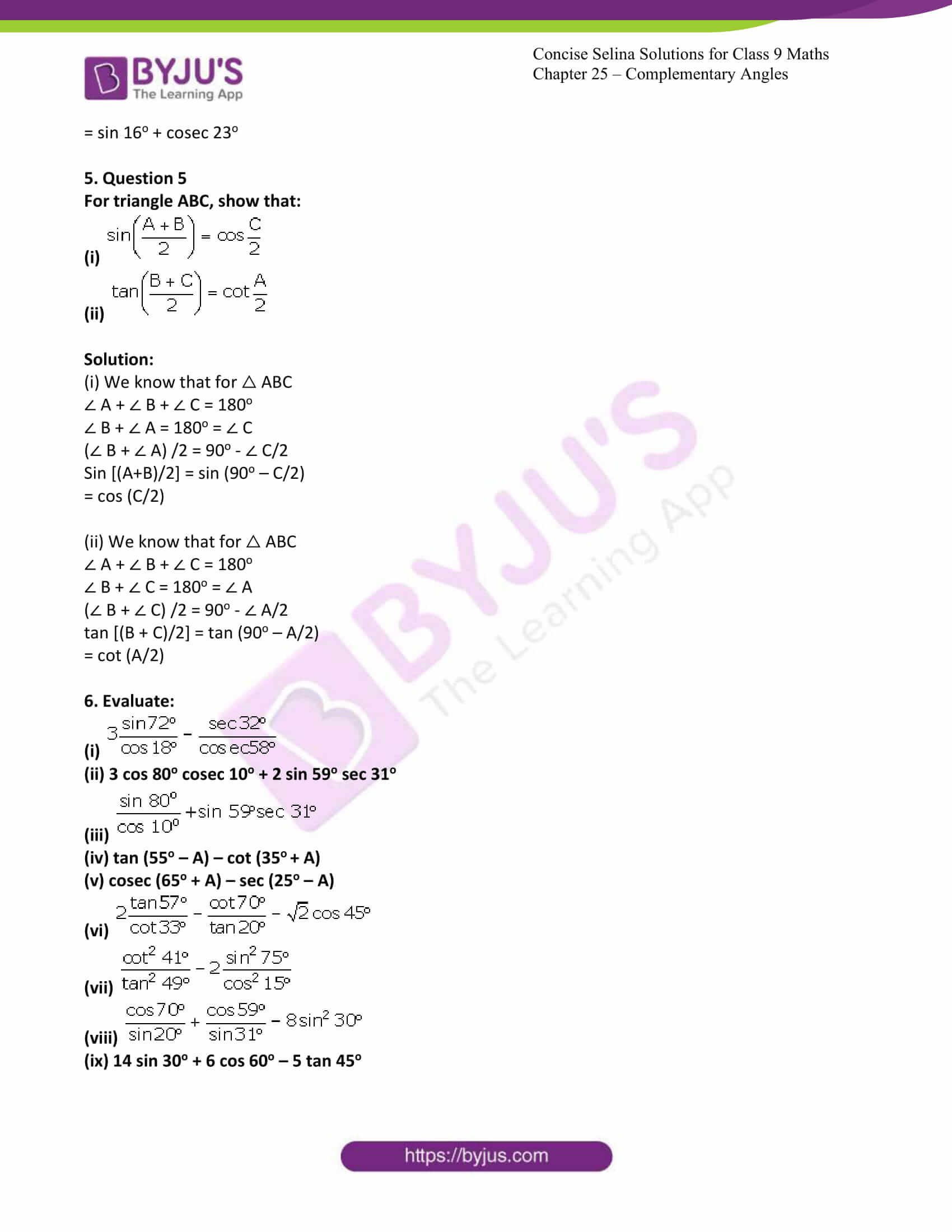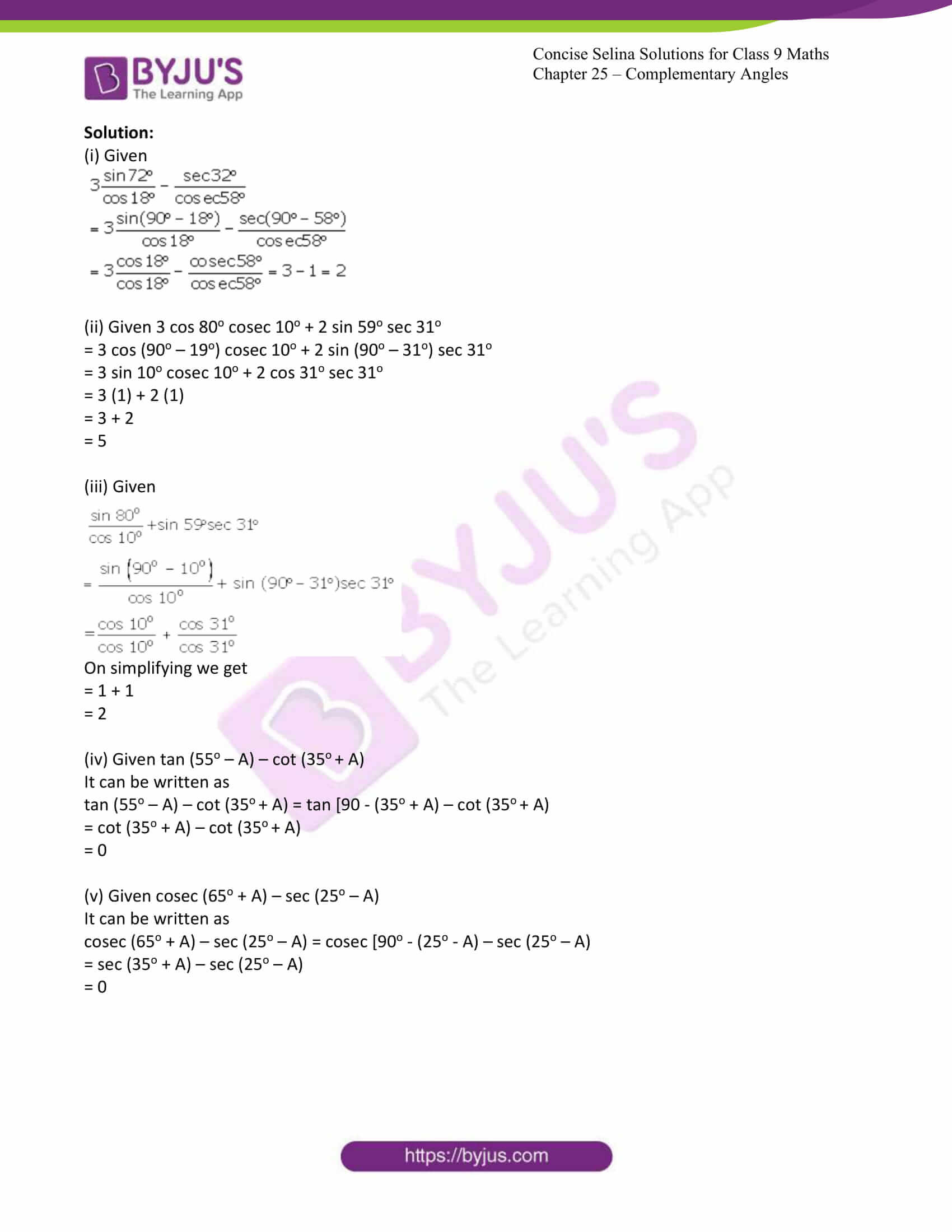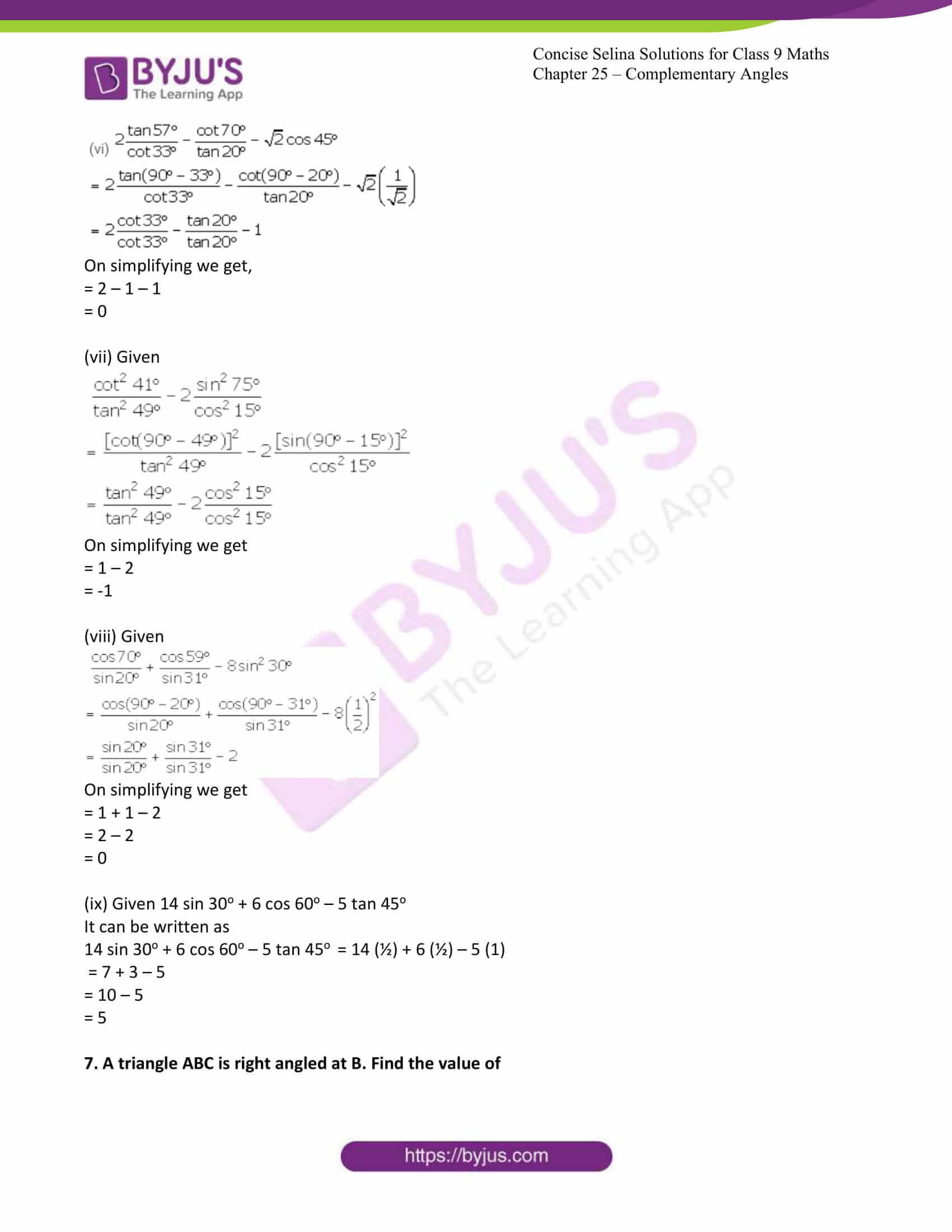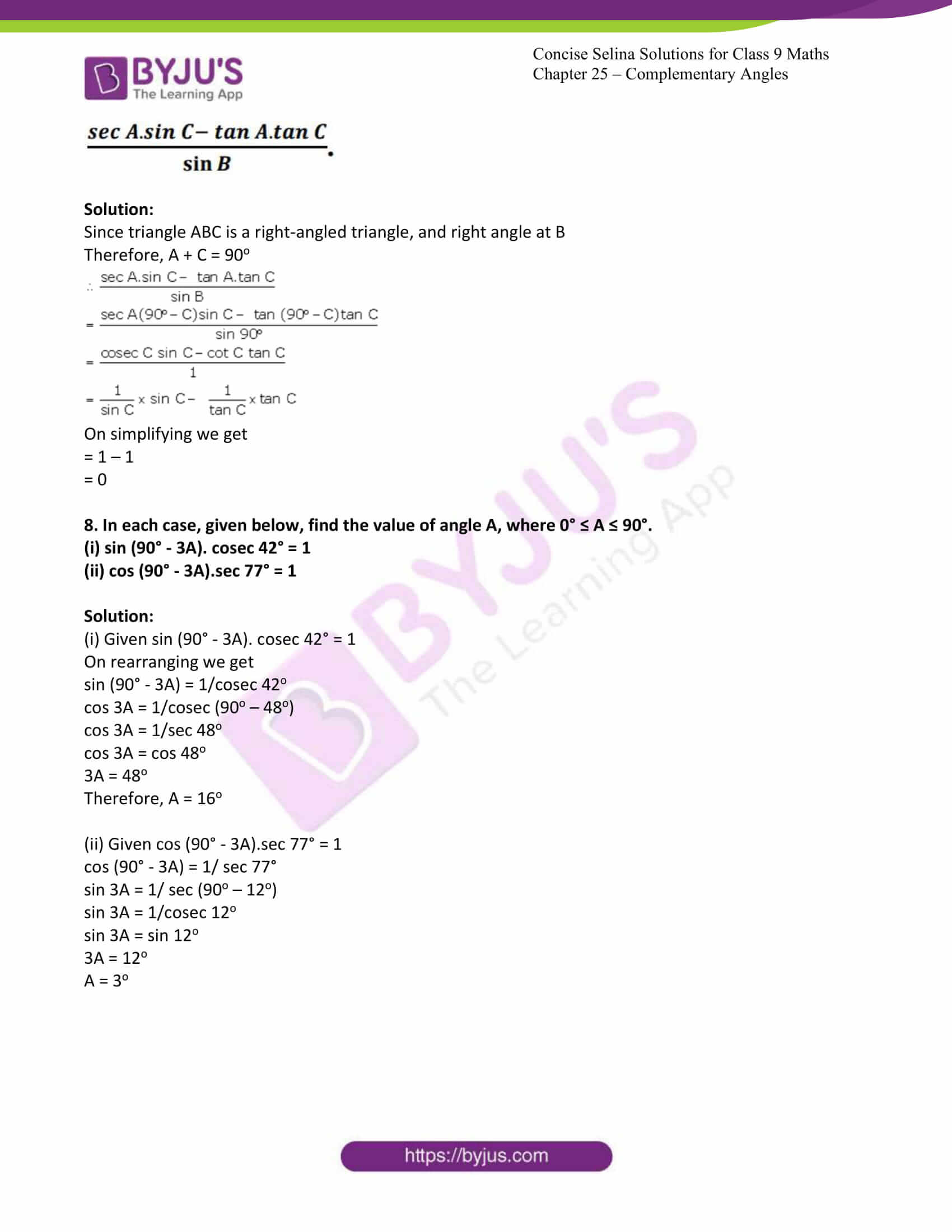Exercise 25

1. Evaluate:

(i) cos 22o/sin 68o

(ii) tan 47o/cot 43o

(iii) sec 75o/cosec 15o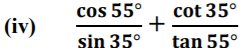(v) sin2 40o – cos2 50o

(vi) sec2 18o – cosec2 72o

(vii) sin 15o cos 15o – cos 75o sin 75o

(viii) sin 42o cos 48o – cos 42o sin 48o

Solution:

(i) According to the question we have,

cos 22o/sin 68o = cos (90o – 68o)/ sin 68o

by using complementary angle identity,

= sin 68o / sin 68o

= 1

(ii) According to the question we have,

tan 47o/cot 43o = tan (90o – 43o)/ cot 43o

by using complementary angle identity,

= cot 43o / cot 43o

= 1

(iii) According to the question we have,

sec 75o/cosec 15o = sec (90o – 15o)/ cosec 15o

by using complementary angle identity,

= cosec 15o / cosec 15o

= 1

(iv) According to the question we have,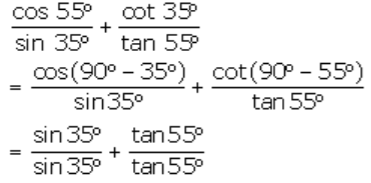= 1 + 1

= 2

(v) sin2 40o – cos2 50o

= sin2 (90o – 50o) – cos2 50o

= cos2 50o – cos2 50o

= 0

(vi) sec2 18o – cosec2 72o

= [sec (90o – 72o)]2 – cosec2 72o

= cosec2 72o – cosec2 72o

= 0

(vii) sin 15o cos 15o – cos 75o sin 75o

= sin (90o – 75o) cos 15o – cos 75o sin (90o – 15o)

= cos 75o cos 15o – cos 75o cos 15o

= 0

(viii) sin 42o sin 48o – cos 42o cos 48o

= sin (90o – 48o) sin 48o – cos (90o – 48o) cos 48o

= cos 48o sin 48o – sin 48o cos 48o

= 0

2. Evaluate:

(i) Sin (90o – A) sin A – cos (90o – A) cos A

(ii) sin2 35o – cos2 55o

(iii)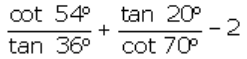(iv)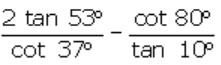(v) cos2 25o – sin265o – tan245o

(vi)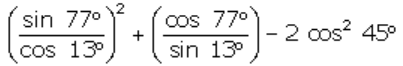Solution:

(i) Given Sin (90o – A) sin A – cos (90o – A) cos A

= cos A sin A – sin A cos A

= 0

(ii) Given sin2 35o – cos2 55o

= sin2 35o – [cos (90o – 35o)]2

= sin2 35o – sin2 35o

= 0

(iii) According to the question we have,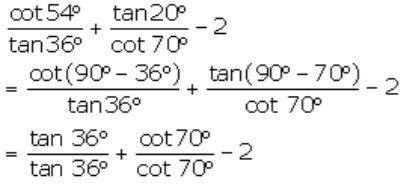On simplifying we get

= 1 + 1 – 2

= 2 – 2

= 0

(iv) According to the question we have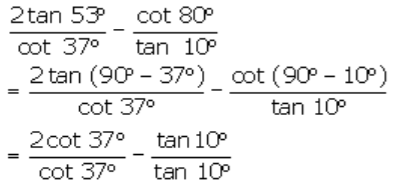On simplifying we get

= 2 – 1

= 1

(v) According to the question we have

Cos2 25o – sin2 65o – tan2 45o

= [cos (90o – 65o)]2 – sin2 65o – (tan2 45o)2

= sin2 65o – sin2 65o – 12

= 0 – 1

= -1

(vi) According to the question we have,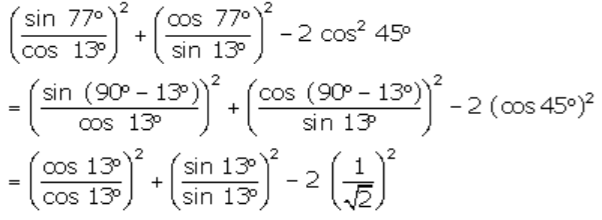On simplifying we get

= 12 + 12 – 2 (½)

= 1 + 1 – 1

= 1

3. Show that:

(i) tan 10° tan 15° tan 75° tan 80° = 1

(ii) sin 42° sec 48° + cos 42° cosec 48° = 2

Solution:

(i) L.H.S.

= tan 10° tan 15° tan 75° tan 80°

= tan (90° – 80°) tan (90° – 75°) tan 75° tan 80°

= cot 80° cot 75 ° tan 75° tan 80°

= (cot 80° tan 80°) (cot 75° tan 75°)

= (1)(1)

= 1

= R.H.S.

(ii) consider LHS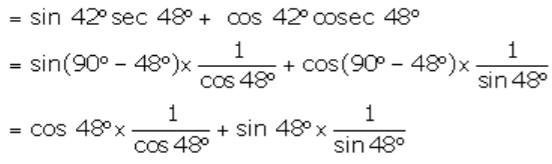On simplifying we get,

= 1 + 1

= 2

= RHS

4. Express each of the following in terms of angles between 0°and 45°:

(i) sin 59°+ tan 63°

(ii) cosec 68°+ cot 72°

(iii) cos 74°+ sec 67°

Solution:

(i) Given sin 59°+ tan 63°

It can be written as

sin 59°+ tan 63° = sin (90 – 31)o + tan(90 – 27)o

= cos 31o + cos 27o

(ii) Given cosec 68°+ cot 72°

It can be written as

cosec 68°+ cot 72° = = cosec (90 – 22)o + cot (90 – 18)o

= sec 22o + tan 18o

(iii) Given cos 74°+ sec 67°

It can be written as

cos 74°+ sec 67° = cos (90 – 16)o + sec(90 – 23)o

= sin 16o + cosec 23o

5. Question 5

For triangle ABC, show that:

(i)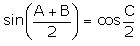(ii)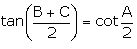Solution:

(i) We know that for △ ABC

∠ A + ∠ B + ∠ C = 180o

∠ B + ∠ A = 180o = ∠ C

(∠ B + ∠ A) /2 = 90o – ∠ C/2

Sin [(A+B)/2] = sin (90o – C/2)

= cos (C/2)

(ii) We know that for △ ABC

∠ A + ∠ B + ∠ C = 180o

∠ B + ∠ C = 180o = ∠ A

(∠ B + ∠ C) /2 = 90o – ∠ A/2

tan [(B + C)/2] = tan (90o – A/2)

= cot (A/2)

6. Evaluate:

(i)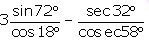(ii) 3 cos 80o cosec 10o + 2 sin 59o sec 31o

(iii)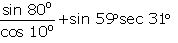(iv) tan (55o – A) – cot (35o + A)

(v) cosec (65o + A) – sec (25o – A)
(vi)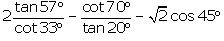(vii)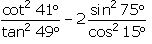(viii)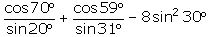(ix) 14 sin 30o + 6 cos 60o – 5 tan 45o

Solution:

(i) Given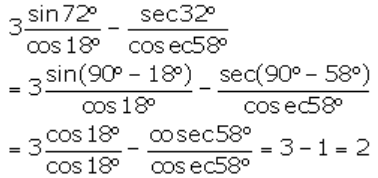(ii) Given 3 cos 80o cosec 10o + 2 sin 59o sec 31o

= 3 cos (90o – 19o) cosec 10o + 2 sin (90o – 31o) sec 31o

= 3 sin 10o cosec 10o + 2 cos 31o sec 31o

= 3 (1) + 2 (1)

= 3 + 2

= 5

(iii) Given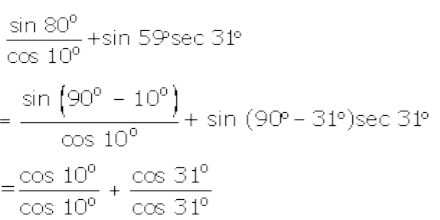On simplifying we get

= 1 + 1

= 2

(iv) Given tan (55o – A) – cot (35o + A)

It can be written as

tan (55o – A) – cot (35o + A) = tan [90 – (35o + A) – cot (35o + A)

= cot (35o + A) – cot (35o + A)

= 0

(v) Given cosec (65o + A) – sec (25o – A)

It can be written as

cosec (65o + A) – sec (25o – A) = cosec [90o – (25o – A) – sec (25o – A)

= sec (35o + A) – sec (25o – A)

= 0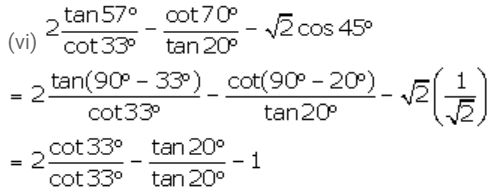On simplifying we get,

= 2 – 1 – 1

= 0

(vii) Given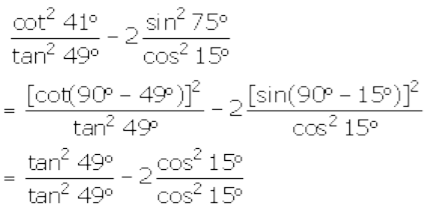On simplifying we get

= 1 – 2

= -1

(viii) Given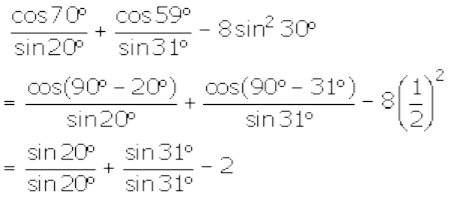On simplifying we get

= 1 + 1 – 2

= 2 – 2

= 0

(ix) Given 14 sin 30o + 6 cos 60o – 5 tan 45o

It can be written as

14 sin 30o + 6 cos 60o – 5 tan 45o = 14 (½) + 6 (½) – 5 (1)

= 7 + 3 – 5

= 10 – 5

= 5

7. A triangle ABC is right angled at B. Find the value of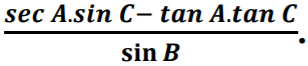Solution:

Since triangle ABC is a right-angled triangle, and right angle at B

Therefore, A + C = 90o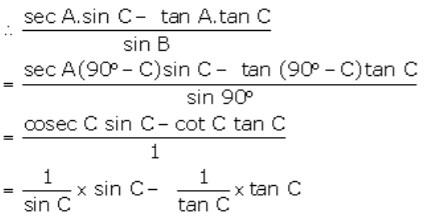On simplifying we get

= 1 – 1

= 0

8. In each case, given below, find the value of angle A, where 0° ≤ A ≤ 90°.

(i) sin (90° – 3A). cosec 42° = 1

(ii) cos (90° – 3A).sec 77° = 1

Solution:

(i) Given sin (90° – 3A). cosec 42° = 1

On rearranging we get

sin (90° – 3A) = 1/cosec 42o

cos 3A = 1/cosec (90o – 48o)

cos 3A = 1/sec 48o

cos 3A = cos 48o

3A = 48o

Therefore, A = 16o

(ii) Given cos (90° – 3A).sec 77° = 1

cos (90° – 3A) = 1/ sec 77°

sin 3A = 1/ sec (90o – 12o)

sin 3A = 1/cosec 12o

sin 3A = sin 12o

3A = 12o

A = 3o

## Selina Solutions for Class 9 Maths Chapter 25- Complementary Angles

Chapter 25, Complementary Angles, is composed of 1 exercise and the solutions given here contain answers to all the questions present in this exercise. Let us have a look at some of the topics that are being discussed in this chapter.

25.1 Introduction

25.2 Concept of trigonometric ratios of complementary angles

25.3 Complementary angles for sine and cosine

## Selina Solutions for Class 9 Maths Chapter 25- Complementary Angles

In Chapters 23 and 24, students were taught about different trigonometric ratios and the evaluation of different trigonometric expressions. On the other hand, chapter 25 discusses and uses the concept of trigonometric ratios of complementary angles along with their applications. Read and learn the 25th chapter of Selina textbook to get up to date with the concepts related to Complementary Angles that are taught in the class. Learn the Selina Solutions for Class 9 effectively to score high in the examination.